# Infinity

In a square with side 18 is inscribed circle, in circle is inscribed next square, again circle and so on to infinity. Calculate the sum of area of all these squares.

Result

S =  648

#### Solution:Leave us a comment of example and its solution (i.e. if it is still somewhat unclear...):

Showing 0 comments:Be the first to comment!#### To solve this example are needed these knowledge from mathematics:

Pythagorean theorem is the base for the right triangle calculator.

## Next similar examples:

1. Square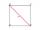Calculate the side of a square with a diagonal measurement 10 cm.
2. Square side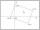Calculate length of side square ABCD with vertex A[0, 0] if diagonal BD lies on line p: -4x -5 =0.
3. Right angled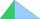From the right triangle with legs 12 cm and 20 cm we built a square with the same content as the triangle. How long will be side of the square?
4. Dog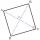Dog is tied to a chain, which is mounted in a corner of the yard. Yard has the shape of a square with a side length of 20 meters. The same long is also dogchain. Are there places in the yard where dog can't reach?
5. SquareCalculate area of the square with diagonal 64 cm.
6. Tree trunkFrom the tree trunk, the diameter at the narrower end is 28 cm, a beam of square cross-section is to be made. Calculate the longest side of the largest possible square cross-section.
7. Square2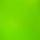Side of the square is a = 6.2 cm, how long is its diagonal?
8. Euklid4Legs of a right triangle have dimensions 244 m and 246 m. Calculate the length of the hypotenuse and the height of this right triangle.
9. CalculationHow much is sum of square root of six and the square root of 225?
10. Geometric progression 48,4√2,4,2√2
11. Geometric progression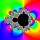Fill 4 numbers between 4 and -12500 to form geometric progression.
12. Euclid2In right triangle ABC with right angle at C is given side a=27 and height v=12. Calculate the perimeter of the triangle.
13. Geometric progression 2There is geometric sequence with a1=5.7 and quotient q=-2.5. Calculate a17.
14. ABS CNCalculate the absolute value of complex number -15-29i.
15. Theorem proveWe want to prove the sentense: If the natural number n is divisible by six, then n is divisible by three. From what assumption we started?
16. Six termsFind the first six terms of the sequence a1 = -3, an = 2 * an-1
17. Vector 7Given vector OA(12,16) and vector OB(4,1). Find vector AB and vector |A|.